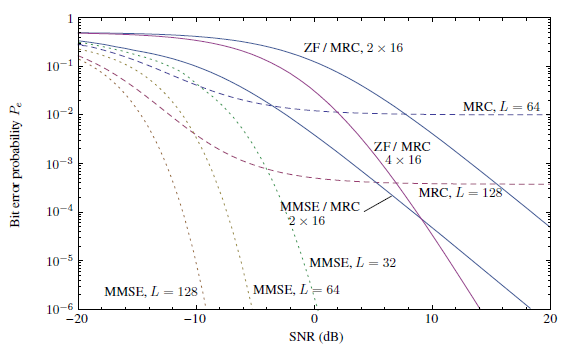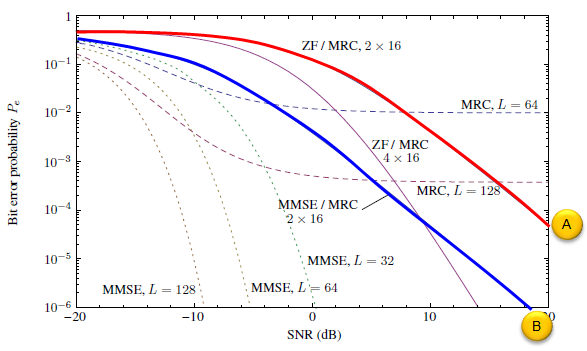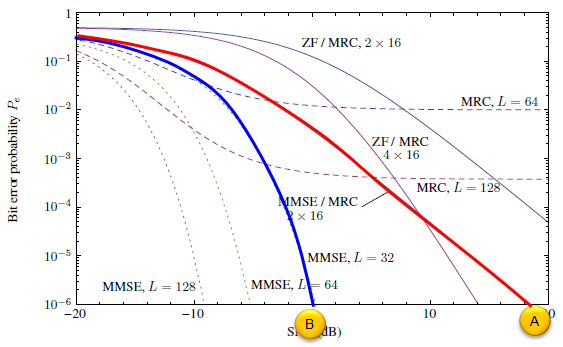5G - Massive MIMO - Reciever Algorithm                             Home : www.sharetechnote.com One of the big questions (research area) in adopting Massive MIMO is what kind of reciever model (algorithm) would be the best fit for this technology. As we see in most case, the first idea tend to come from existing technologies and the most common candidates are MRC(Maximum Ratio Combining), ZF (Zero Force) and MMSE(Minimized Mean Square Error). You can have general introduction of these reciever technology in ZF and MMSE page. Those pages are only for general introduction, not specifically for Massive MIMO, but you may have some big picture from those pages if you are new to this area.   The efficiency of Massive MIMO Reciever Model    Now let's think of what would be the best candidate  for Massive MIMO technology. Of course, there would be no clear answer to this question. The answer might be different depending on how you define the world 'the best' candidate.   I think a single graph from  Nutaq whitepaper titled "Two-Layer Linear Processing for Massive MIMO on the Titan MIMO Platform" (See Reference ) would give you a lot of information or idea when you try to chose reciever algorithm. The graph looks as follows.Whenever you are given this kind of graph, it would not make much sense to to you (or would not give you much idea) unless you take very close look. (If you are new to this area, the meaning of graph itself would not be clear .. I will post another introductary page to interpret this kind of performance graph later).   Now let's look more deeply into the graph and see what this graph really try to tell.   First, let take a look at only two curve (marked in red(A) and blue(B) in the following graph). (A) use 64 antenna and (B) use 128 antenna, and both (A) and (B) use the same algorithm(MRC). You see (B) shows better performance. This is understandable. But you see you would not achieve good enough performance regardless of how many antenna you use. Even with 128 antenna, the performance (error rate) does not improve any further from SNR of roughly 6 dB.Now, let take a look at following three curves (marked in red(A), blue(B) and green(C) in the following graph). (A) use 32 antenna, (B) use 64 antenna and (C) uses 128. (A),(B) and (C) use the same algorithm (MMSE). You see (B) shows better performance than (A) and (C) performs better than (B). This is understandable. also it seems that these are good enough to use in real life. It is true if you only think of performance. However, if you look into the MMSE algorithm in MMSE Introduction page, you would notice that this algorithm requires a lot of matrix calculation especially 'matrix inverse' operation. This matrix operation would take exponentially larger processing power (memory, calculation time etc) as the size of the matrix grow larger. So it might become a little bit difficult (or inefficient in terms of processing power and complexity of implementation).Now, let take a look at another set of curves (marked in red(A) and blue(B) in the following graph). Both (A) and (B) uses the same number of Antenna (32 antenna) but they use different algorithms. One thing you should notice is that these are not using single algorithm for each case, they are using a combined algorithm. In (A), it uses a combined algorithm of ZF and MRC and in (B), it uses a combined algorithm of MMSE and MRC. (I will post another separate page to show the details of how these two algorithm are combined). Here, you only have to see MMSE+MRC (B) shows better performance than ZF+MRC (A).Now let's compare following two curves. As you see, These two cases are using the same number of antenna (128) but using different reciever algorithm( (A) = MRC, (B) = MMSE). You would see that the curve (B) shows much better performance.Now let's compare another set of curves. As you see, These two cases are using the same number of antenna (32) but using different reciever algorithm( (A) = MRC+MMSE, (B) = MMSE). You would see that the curve (B) shows much better performance. Which algorithm would you pick for your system ? If you think only of performance itself, you would pick (B), but if you consider the required processing power and complexity, (A) can be a choice as well (This is what Nutaq suggests in the whitepaper. Of course, the final decision is all yours :)Reference   S�bastien Roy Dept. of Electrical and Computer Engineering Universit� de Sherbrooke e-mail: s.roy17@usherbrooke.ca    5G NOW. D3.1 5G Waveform Candidate Selection    Massive MIMO and Small Cells : Improving Energy Efficiency by Optimal Soft-Cell Coordination Emil Bjornson, Marios Kountouris and Merouane Debbah Alcatel-Lucent Chair on Flexible Radio, SUPELEC, Gif-sur-Yvette, France Department of Telecommunications, SUPELEC, Gif-sur-Yvette, France ACCESS Linnaeus Center, Signal Processing Lab, KTH Royal Institue of Technology, Stockholm, Sweden      Massive MIMO for Next Generation Wireless Systems Erik G. Larson, ISY, Linkoping University, Sweden Ove Edfors, Lund University, Sweden Fredrik Tufvesson, Lund University, Sweden Thomas L. Marzetta, Bell Labs, Alcatel-Lucent, USA    Scaling up MIMO : Opportunities and Challenges with Very Large Arrays Fredrik Rusek, Dept. of Electrical and Information Technology, Lund University, Lund, Sweden Daniel Persson, Dept. of Electrical Engineering (ISY), Linkoping University, Sweden Buon Kiong Lau, Dept. of Electrical and Information Technology, Lund University, Lund, Sweden Erik G. Larsson, Dept. of Electrical Engineering (ISY), Linkoping University, Sweden Thomas L. Marzetta, Bell Laboratories, Alcatel-Lucent, Murray Hill, NJ Ove Edfors, Dept. of Electrical and Information Technology, Lund University, Lund, Sweden Fredrik Tufvesson, Dept. of Electrical and Information Technology, Lund University, Lund, Sweden